# 2019算法岗阿里笔试编程题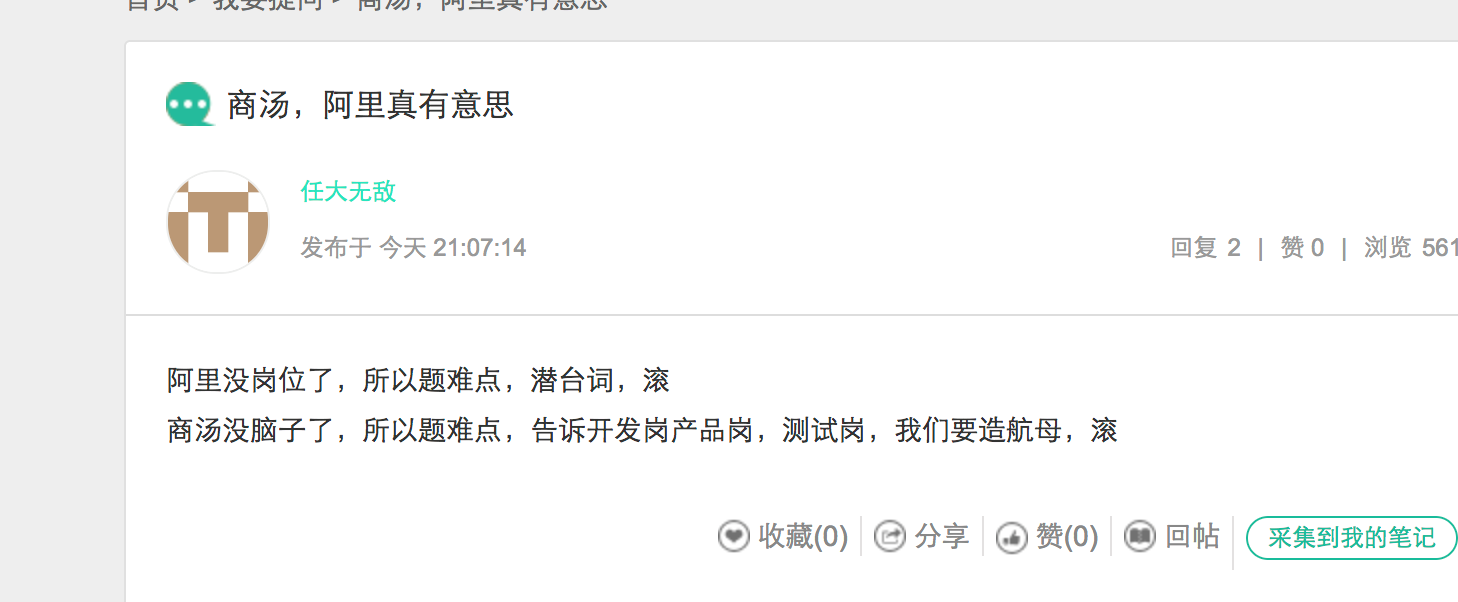## 阿里：编程题1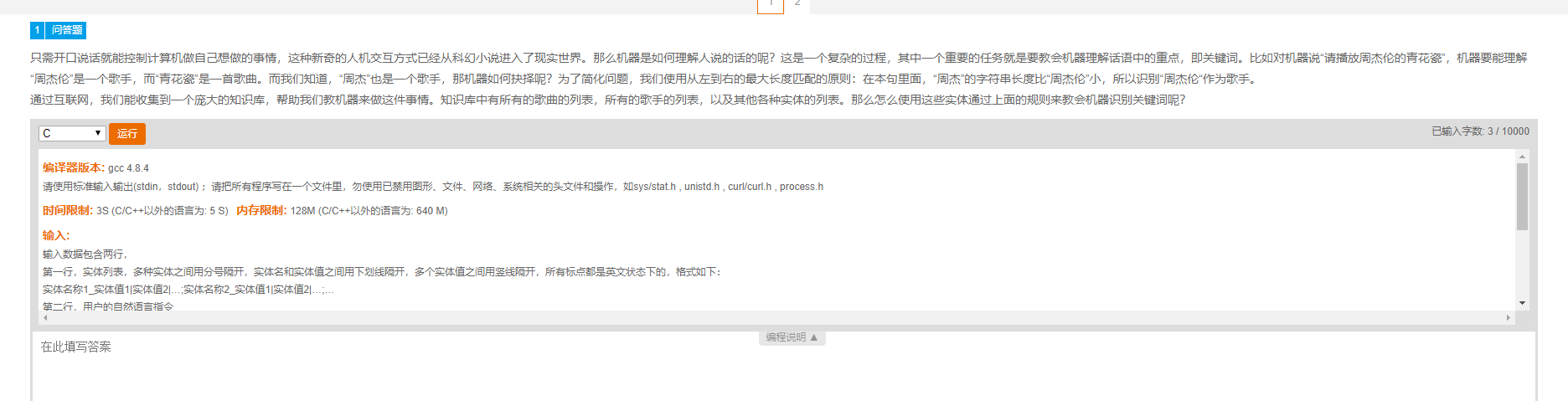singer_周杰|周杰伦|刘德华|王力宏;song_冰雨|北京欢迎你|七里香;actor_周杰伦|孙俪


def Solve(inputs, dicts):
res = ""
i = len(inputs) - 1
while i>=0:
j = i - 1
s = inputs[j:i+1]
while j>=0 and s not in dicts:
j -= 1
s = inputs[j:i+1]

if s in dicts:
res = ' ' + s + '/' + dicts[s] + ' '+ res
i  = j-1
else:
res = inputs[i] + res
i -= 1
return res

import sys
if __name__ == '__main__':
ss = input().split(';')
dicts = {}
names = []

for s in ss:
s = s.split('_')
s = s.split('|')
for i in range(len(s)):
key = s[i]
if key not in dicts:
dicts[key] = s
else:
dicts[key] = dicts[key] + ',' + s
names += s

strings = input()

res = Solve(strings, dicts)
sys.stdout.write(res + '\n')

singer_zj|zjl;song_by|qlx;actor_zjl|sl
qbfzjldqlxgwt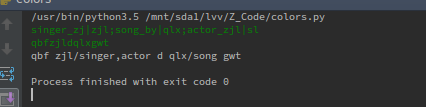## 阿里编程第二题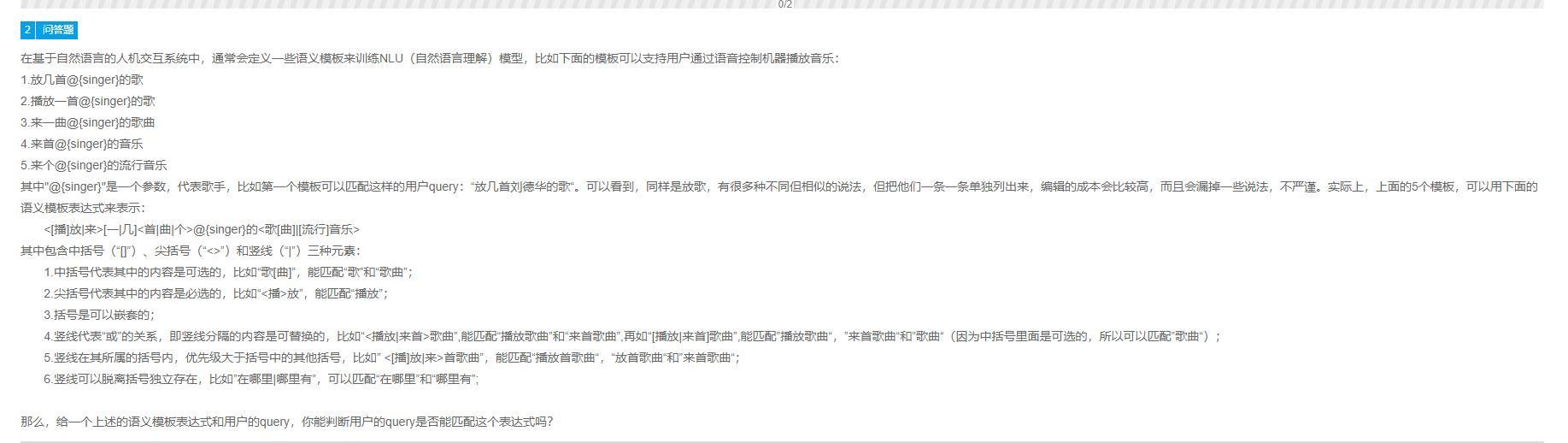<[播]放|来>[一|几]<首|曲|个>@{singer}的<歌[曲]|[流行]音乐>

0

def inprocess(strings):
dicts = {'<':'>', '>':'<', '[':']', ']':'[' }

flag = []
i = 0
res = []
start = 0
# split inputs to many strings
while i <len(strings):
s = strings[i]
if s=='<' or s=='[':
flag.append(s)
if strings[start]=='@':
res.append(strings[start:i])
start = i

elif flag and s==dicts[flag[-1]]:
tmp = flag.pop()
if flag:
continue
else:
res.append(strings[start+1:i])
start = i+1
del tmp

i += 1

# process the res
collect = []
for id, lists in enumerate(res):
if '|' not in lists:
collect.append(lists)
continue

lists = lists.split('|')
for s in lists:
i = 0
# while i < len(s):
if s[i]=='[':
collect.append(s[i+1:s.index(']')])
i = s.index(']')+1
if i<len(s):
collect.append(collect[-1]+s[i:])

elif '[' not in s:
collect.append(s)
for c in res:
print(c)
print(collect)
return collect

def testing(qurry, collect):
i = 0
while i<len(qurry):
c = qurry[i]
if c in collect:
collect = collect[collect.index(c)+1:]
elif c=='@':
if qurry[i:qurry.index('}')+2] in collect:
i = qurry.index('}') + 1
else:
return 0
else:
return 0
i += 1
return 1

if __name__ == '__main__':
inputs = "<[B]F|L>[Y|J]<S|Q|G>@{singer}G<[Q]|[LX]YL>"
res = inprocess(inputs)

qurry = "LJS@{singer}GLXGQ"

print(testing(qurry, res))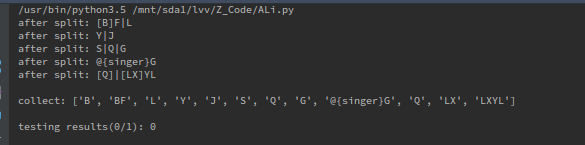03-032万+

#### 阿里2019届内推笔试编程题（算法岗+开发岗）04-153963

#### 2019阿里暑期实习笔试编程题

09-043610

#### 记某一次阿里面试的实时笔试题

05-146226

#### 阿里巴巴在线笔试编程题2题

08-30190

#### 本吊阿里在线笔试题目

08-26

09-171272

#### 2020阿里笔试编程题

08-24109

#### 阿里笔试算法题之词的匹配

03-121万+

#### 阿里巴巴历年在线笔试题汇总

04-131555

#### 阿里2019年实习春招算法机器学习笔试编程题及解答

04-10660

#### 阿里2019算法实习生题目

05-082313

#### 一些算法编程题整理

03-066万+

#### 历年阿里面试题汇总（2017年不断更新中）

08-304万+

#### 阿里笔试题(2015)持续更新中

08-26189

#### 阿里算法岗

03-097942

#### 2018阿里巴巴算法岗位实习面试总结（朋友的面试）©️2020 CSDN 皮肤主题: 大白 设计师: CSDN官方博客点击重新获取扫码支付1.余额是钱包充值的虚拟货币，按照1:1的比例进行支付金额的抵扣。
2.余额无法直接购买下载，可以购买VIP、C币套餐、付费专栏及课程。余额充值